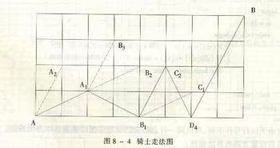### 1.A:六皇后

#### 题目描述#### 样例输入

``````6
``````

#### 样例输出

``````2 4 6 1 3 5
3 6 2 5 1 4
4 1 5 2 6 3
4
``````

#### 参考程序(自己打的)

``````#include<iostream>
using namespace std;
int count = 0;
int chess={0};
int notDanger(int row,int col ){
int i,k;
for(i=0;i<6;i++){
if(chess[i][col]==1)
return 0;
}
for(i=row,k=col;i>=0&&k>=0;i--,k--)
if(chess[i][k]==1)
return 0;

for(i=row,k=col;i>=0&&k<6;i--,k++)
if(chess[i][k]==1)
return 0;

return 1;
}

void Print(){
int i,j;
for(i=0;i<6;i++){
for(j=0;j<6;j++){
if(chess[i][j]==1)
cout<<j+1<<" ";
}
}
cout<<endl;
}
void EightQueen( int row ){
int col;
if( row>5 )                       {
Print();
count++;
return ;}
``````

### 2.B:东南西北

#### 样例输入

``````1 1
2 2
5
E
N
W
W
N
``````

#### 样例输出

``````2
``````

#### 参考程序

``````#include <iostream>
using namespace std;
int x,y,x1,y1,n,s=0;
char a;
int l=0;
int main()
{
cin>>x>>y>>x1>>y1;
cin>>n;
if(x==x1&&y==y1){cout<<'0';return 0;}
for(int i=1;i<=n;i++)
{
cin>>a;
if(x1-x>0&&a=='E')x++,s++;
else if(x1-x<0&&a=='W')x--,s++;
if(y1-y>0&&a=='N')y++,s++;
else if(y1-y<0&&a=='S')y--,s++;
}
if(x==x1&&y==y1)cout<<s;
else cout<<"-1";
return 0;
}
``````

### 3.C:跳马

#### 题目描述#### 样例输入

``````4 8
``````

#### 样例输出

``````37
``````

#### 参考程序

``````#include <stdio.h>
#include <queue>
using namespace std;
int dx= {1, 2, 1, 2};
int dy= {2, 1, -2, -1};//四种走法
int ways=0;//记录路线条数

struct NODE {
int x, y;
};//记录路线上的点的坐标

bool Valid (NODE h, int m, int n) {
if ((h.x<=m)&&(h.y>=1)&&(h.y)<=n)
return 1;
else
return 0;
}//判断下的这步棋是否符合规则

bool bfs (NODE s, int m, int n) {
queue<NODE>q;//建立路线队列
NODE now, next;//用于记录当前棋和进入下一步的棋
q.push(s);//起点入列
while (!q.empty()) {
now = q.front();
q.pop();//取出当前棋子并出列
if ((now.x==m)&&(now.y==n)) {
ways++;
continue;
}//判断是否走到终点
for ( int i=0; i<4; i++) {
next.x=now.x+dx[i];
next.y=now.y+dy[i];//走出四种走法
if (Valid(next,m, n))
q.push(next);//如果有效则入列
}
}
}

int main() {
int m, n;
scanf ("%d %d", &m, &n);
NODE s;
s= {1,1};//起点
bfs (s, n, m);//开始搜索
printf ("%d\n", ways);
return 0;
}
``````

### 4.D:奇怪的电梯

#### 题目描述

(K
1
=3,K
2
=3,…)，从11楼开始。在1楼，按“上”可以到4楼，按“下”是不起作用的，因为没有-2楼。那么，从A楼到B楼至少要按几次按钮呢？

#### 样例输入

``````5 1 5
3 3 1 2 5
``````

#### 样例输出

``````3
``````

#### 参考程序

``````#include <iostream>
#include <vector>
#include <queue>

using namespace std;

int main()
{
queue<int> q;  //BFS 实现用队列
vector<int> v; //保存每层按钮上的数值
vector<int> f; //到达每层最少的按键次数
vector<bool> flag;//标记值，表示每层是否被访问过，true代表访问过
int n;
int start, end;//开始层和结束层
int tmp;
cin >> n >> start >> end;
v.push_back(0);//方便起见，下标从1开始
f.push_back(0);
flag.push_back(false);
for (int  i = 1; i <= n; i++)
{
cin >> tmp;
v.push_back(tmp);
if(i == start)
f.push_back(0);//初始化,start层开始为按0次,其余层为-1次
else
f.push_back(-1);
flag.push_back(false);
}
q.push(start);

while (!q.empty()&& !flag.at(q.front()))//队列不为空，切第q.front()层没有被访问过
{
flag.at(q.front()) = true;
int next = q.front() + v.at(q.front());
if (next <= n) //上楼
{
q.push(next);
f.at(next) = f.at(q.front()) + 1;//记下到达每层最少的按键次数
if (next == end) //找到end层，退出while循环
{
break;
}
}
next = q.front() - v.at(q.front());
if (next >= 1)//下楼
{
q.push(next);
f.at(next) = f.at(q.front()) + 1;
if (next == end)//找到end层，退出while循环
{
break;
}
}
q.pop();
}
cout << f.at(end) << endl;

return 0;
}
``````